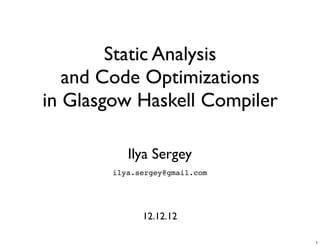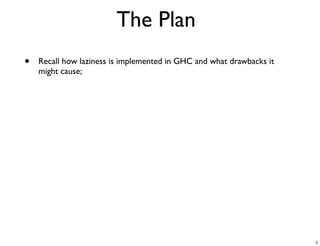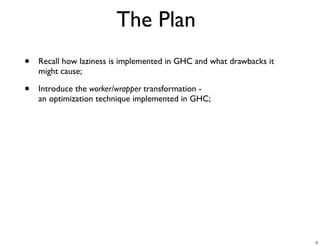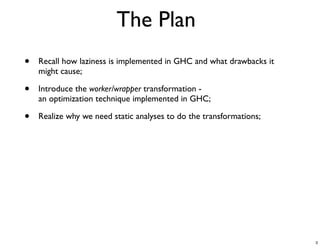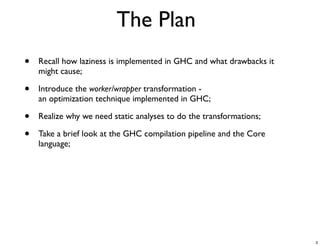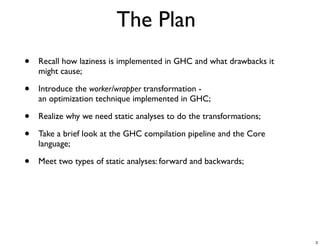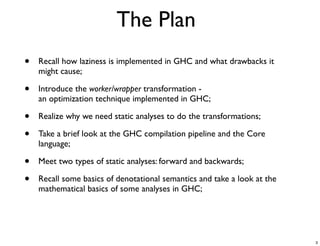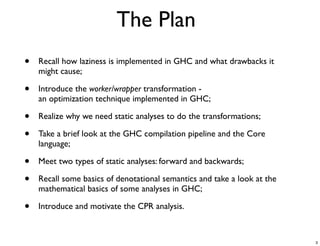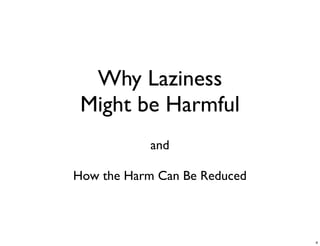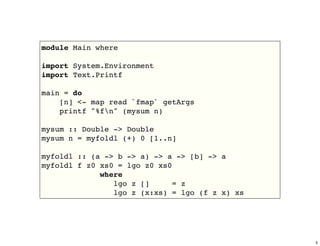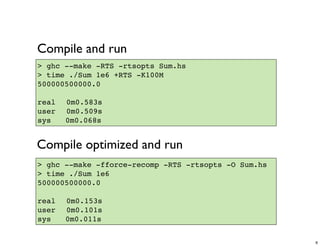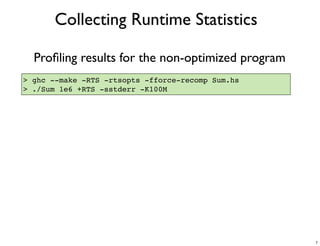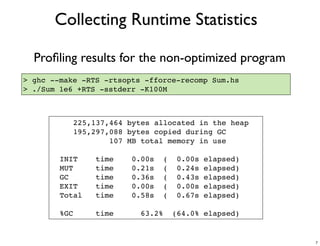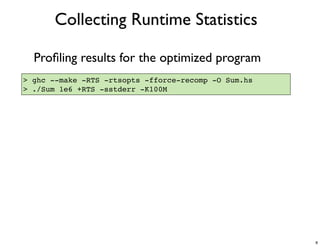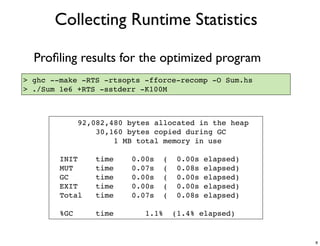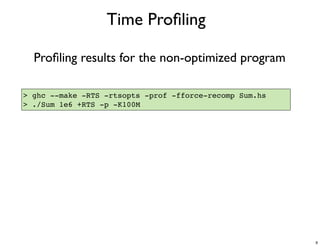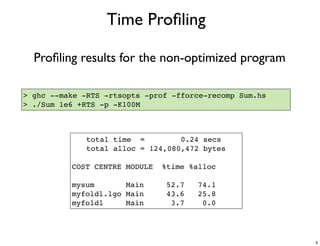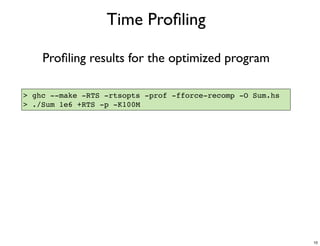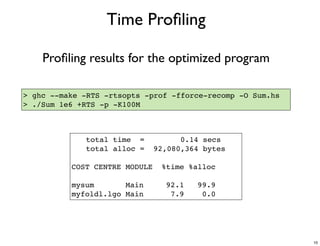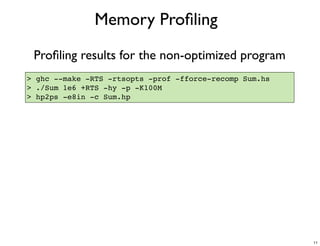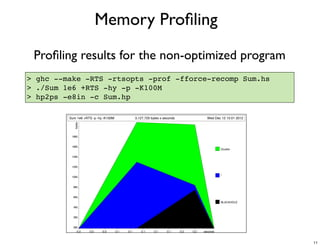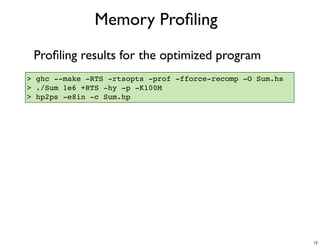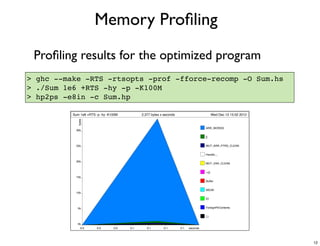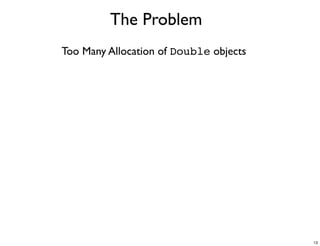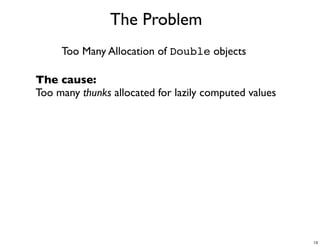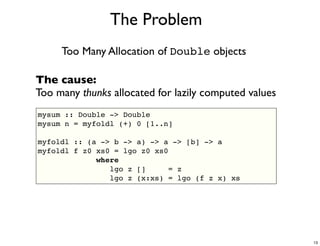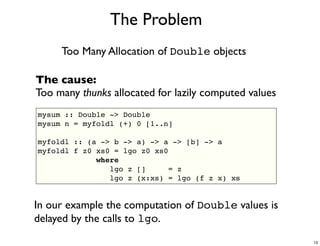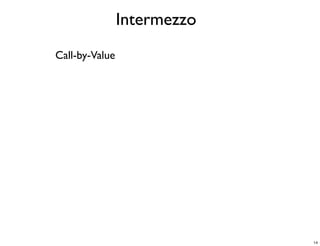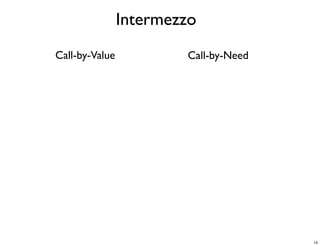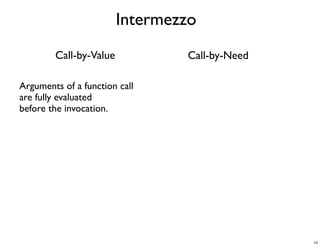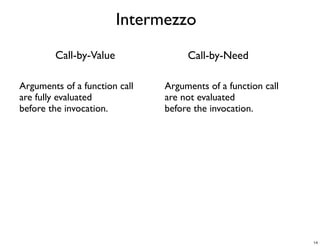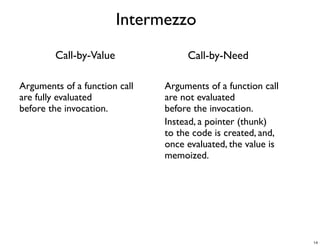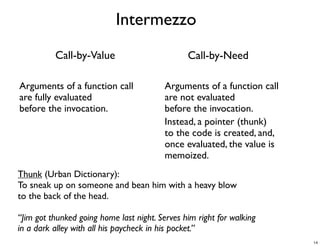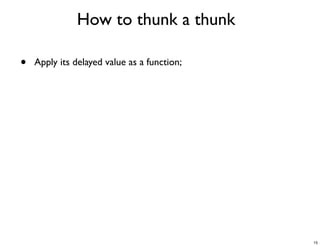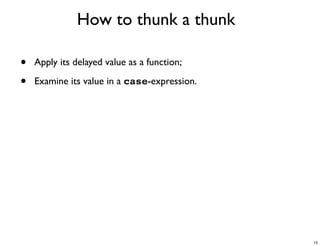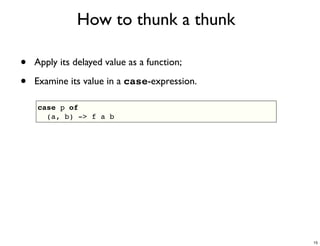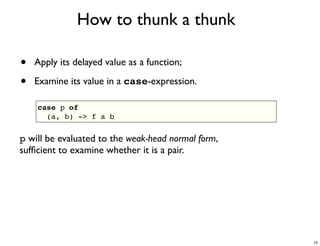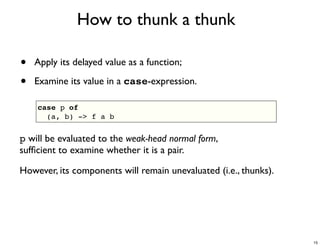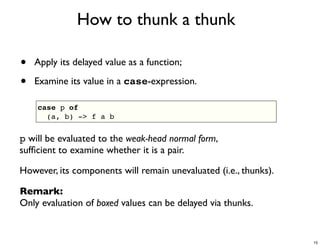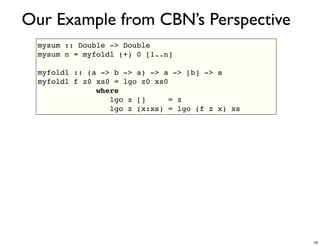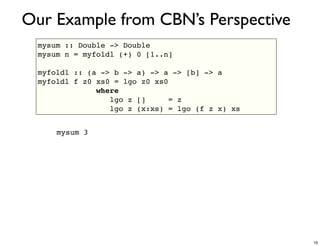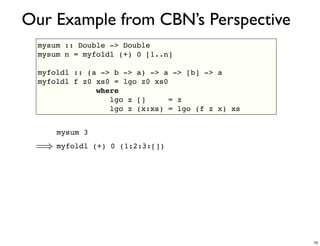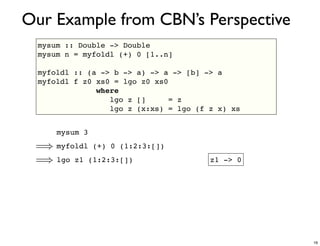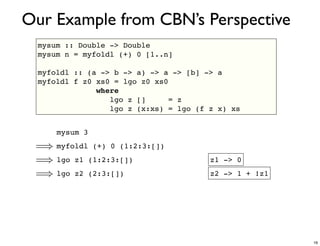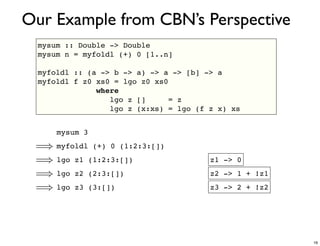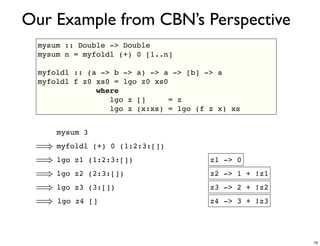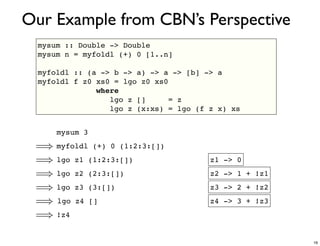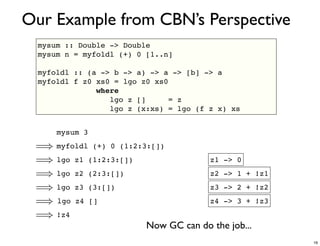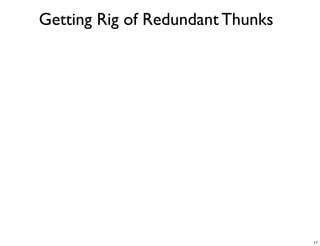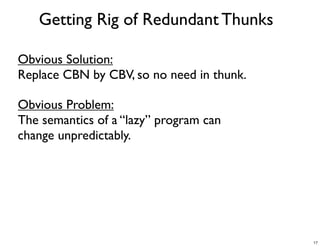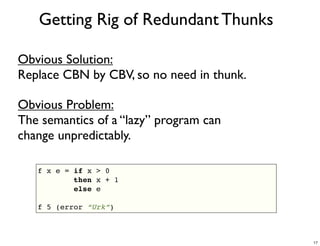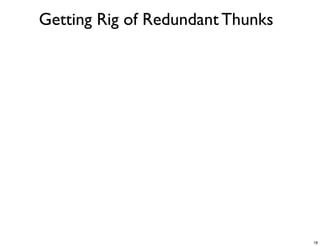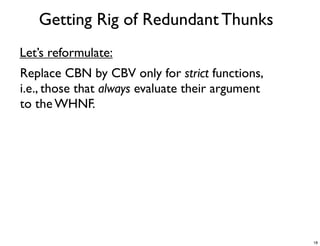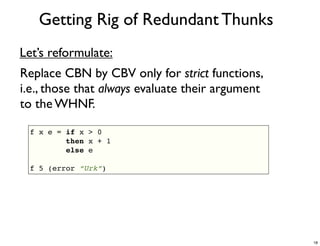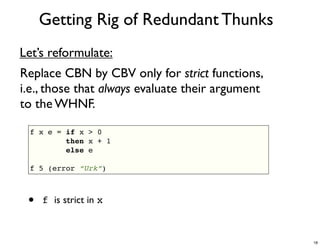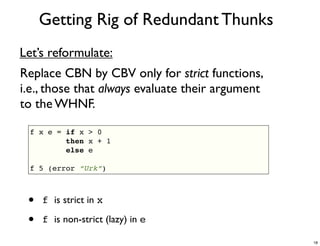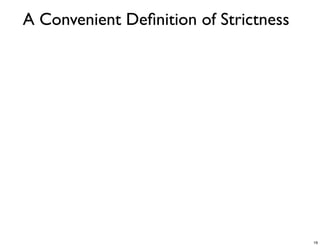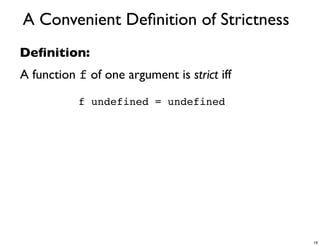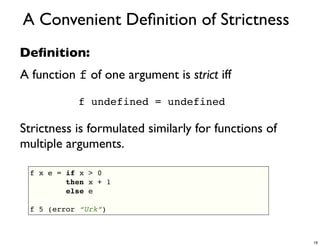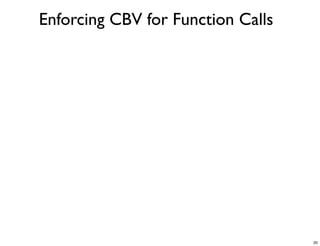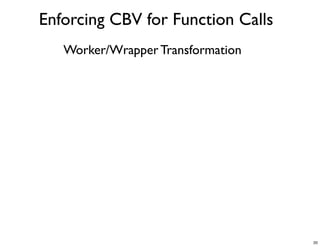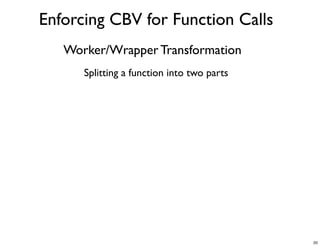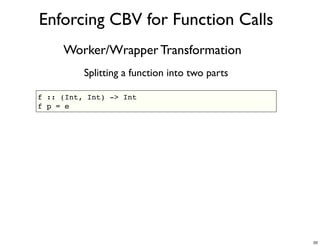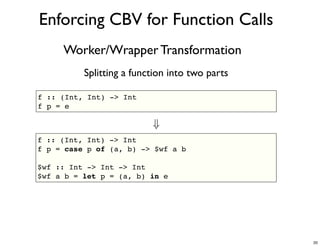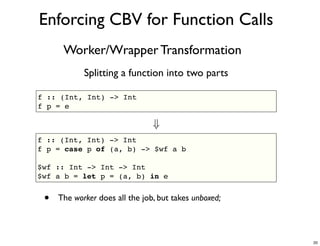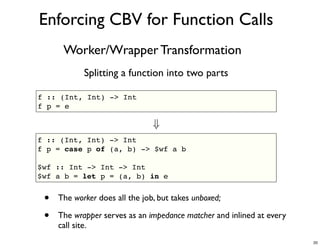1 of 74

### Static Analysis and Code Optimizations in Glasgow Haskell Compiler

1. Static Analysis and Code Optimizations in Glasgow Haskell Compiler Ilya Sergey ilya.sergey@gmail.com 12.12.12 1
2. The Goal Discuss what happens when we run ghc -O MyProgram.hs 2
3. The Plan • Recall how laziness is implemented in GHC and what drawbacks it might cause; • Introduce the worker/wrapper transformation - an optimization technique implemented in GHC; • Realize why we need static analysis to do the transformations; • Take a brief look at the GHC compilation pipeline and the Core language; • Meet two types of static analysis: forward and backwards; • Recall some basics of denotational semantics and take a look at the mathematical basics of some analyses in GHC; • Introduce and motivate the CPR analysis. 3
4. Why Laziness Might be Harmful and How the Harm Can Be Reduced 4
5. module Main where import System.Environment import Text.Printf main = do [n] <- map read `fmap` getArgs printf "%fn" (mysum n) mysum :: Double -> Double mysum n = myfoldl (+) 0 [1..n] myfoldl :: (a -> b -> a) -> a -> [b] -> a myfoldl f z0 xs0 = lgo z0 xs0 where lgo z [] = z lgo z (x:xs) = lgo (f z x) xs 5
6. Compile and run > ghc --make -RTS -rtsopts Sum.hs > time ./Sum 1e6 +RTS -K100M 500000500000.0 real! 0m0.583s user! 0m0.509s sys! 0m0.068s Compile optimized and run > ghc --make -fforce-recomp -RTS -rtsopts -O Sum.hs > time ./Sum 1e6 500000500000.0 real! 0m0.153s user! 0m0.101s sys! 0m0.011s 6
7. Collecting Runtime Statistics Proﬁling results for the non-optimized program > ghc --make -RTS -rtsopts -fforce-recomp Sum.hs > ./Sum 1e6 +RTS -sstderr -K100M 225,137,464 bytes allocated in the heap 195,297,088 bytes copied during GC 107 MB total memory in use INIT time 0.00s ( 0.00s elapsed) MUT time 0.21s ( 0.24s elapsed) GC time 0.36s ( 0.43s elapsed) EXIT time 0.00s ( 0.00s elapsed) Total time 0.58s ( 0.67s elapsed) %GC time 63.2% (64.0% elapsed) 7
8. Collecting Runtime Statistics Proﬁling results for the optimized program > ghc --make -RTS -rtsopts -fforce-recomp -O Sum.hs > ./Sum 1e6 +RTS -sstderr -K100M 92,082,480 bytes allocated in the heap 30,160 bytes copied during GC 1 MB total memory in use INIT time 0.00s ( 0.00s elapsed) MUT time 0.07s ( 0.08s elapsed) GC time 0.00s ( 0.00s elapsed) EXIT time 0.00s ( 0.00s elapsed) Total time 0.07s ( 0.08s elapsed) %GC time 1.1% (1.4% elapsed) 8
9. Time Proﬁling Proﬁling results for the non-optimized program > ghc --make -RTS -rtsopts -prof -fforce-recomp Sum.hs > ./Sum 1e6 +RTS -p -K100M ! total time = 0.24 secs ! total alloc = 124,080,472 bytes COST CENTRE MODULE %time %alloc mysum Main 52.7 74.1 myfoldl.lgo Main 43.6 25.8 myfoldl Main 3.7 0.0 9
10. Time Proﬁling Proﬁling results for the optimized program > ghc --make -RTS -rtsopts -prof -fforce-recomp -O Sum.hs > ./Sum 1e6 +RTS -p -K100M ! total time = 0.14 secs ! total alloc = 92,080,364 bytes COST CENTRE MODULE %time %alloc mysum Main 92.1 99.9 myfoldl.lgo Main 7.9 0.0 10
11. Memory Proﬁling Proﬁling results for the non-optimized program > ghc --make -RTS -rtsopts -prof -fforce-recomp Sum.hs > ./Sum 1e6 +RTS -hy -p -K100M > hp2ps -e8in -c Sum.hp Sum 1e6 +RTS -p -hy -K100M 3,127,720 bytes x seconds Wed Dec 12 15:01 2012 bytes 18M 16M Double 14M 12M 10M * 8M 6M BLACKHOLE 4M 2M 0M 0.0 0.0 0.0 0.1 0.1 0.1 0.1 0.1 0.2 0.2 seconds 11
12. Memory Proﬁling Proﬁling results for the optimized program > ghc --make -RTS -rtsopts -prof -fforce-recomp -O Sum.hs > ./Sum 1e6 +RTS -hy -p -K100M > hp2ps -e8in -c Sum.hp Sum 1e6 +RTS -p -hy -K100M 2,377 bytes x seconds Wed Dec 12 15:02 2012 bytes ARR_WORDS 30k [] 25k MUT_ARR_PTRS_CLEAN Handle__ 20k MUT_VAR_CLEAN ->[] 15k Buffer WEAK 10k IO 5k ForeignPtrContents (,) 0k 0.0 0.0 0.0 0.1 0.1 0.1 0.1 seconds 12
13. The Problem Too Many Allocation of Double objects The cause: Too many thunks allocated for lazily computed values mysum :: Double -> Double mysum n = myfoldl (+) 0 [1..n] myfoldl :: (a -> b -> a) -> a -> [b] -> a myfoldl f z0 xs0 = lgo z0 xs0 where lgo z [] = z lgo z (x:xs) = lgo (f z x) xs In our example the computation of Double values is delayed by the calls to lgo. 13
14. Intermezzo Call-by-Value Call-by-Need Arguments of a function call Arguments of a function call are fully evaluated are not evaluated before the invocation. before the invocation. Instead, a pointer (thunk) to the code is created, and, once evaluated, the value is memoized. Thunk (Urban Dictionary): To sneak up on someone and bean him with a heavy blow to the back of the head. “Jim got thunked going home last night. Serves him right for walking in a dark alley with all his paycheck in his pocket.” 14
15. How to thunk a thunk • Apply its delayed value as a function; • Examine its value in a case-expression. case p of (a, b) -> f a b p will be evaluated to the weak-head normal form, sufﬁcient to examine whether it is a pair. However, its components will remain unevaluated (i.e., thunks). Remark: Only evaluation of boxed values can be delayed via thunks. 15
16. Our Example from CBN’s Perspective mysum :: Double -> Double mysum n = myfoldl (+) 0 [1..n] myfoldl :: (a -> b -> a) -> a -> [b] -> a myfoldl f z0 xs0 = lgo z0 xs0 where lgo z [] = z lgo z (x:xs) = lgo (f z x) xs mysum 3 =) myfoldl (+) 0 (1:2:3:[]) =) lgo z1 (1:2:3:[]) z1 -> 0 =) lgo z2 (2:3:[]) z2 -> 1 + !z1 =) lgo z3 (3:[]) z3 -> 2 + !z2 =) lgo z4 [] z4 -> 3 + !z3 =) !z4 Now GC can do the job... 16
17. Getting Rid of Redundant Thunks Obvious Solution: Replace CBN by CBV, so no need in thunk. Obvious Problem: The semantics of a “lazy” program can change unpredictably. f x e = if x > 0 then x + 1 else e f 5 (error “Urk”) 17
18. Getting Rid of Redundant Thunks Let’s reformulate: Replace CBN by CBV only for strict functions, i.e., those that always evaluate their argument to the WHNF. f x e = if x > 0 then x + 1 else e f 5 (error “Urk”) • f is strict in x • f is non-strict (lazy) in e 18
19. A Convenient Deﬁnition of Strictness Deﬁnition: A function f of one argument is strict iff f undefined = undefined Strictness is formulated similarly for functions of multiple arguments. f x e = if x > 0 then x + 1 else e f 5 (error “Urk”) 19
20. Enforcing CBV for Function Calls Worker/Wrapper Transformation Splitting a function into two parts f :: (Int, Int) -> Int f p = e + f :: (Int, Int) -> Int f p = case p of (a, b) -> \$wf a b \$wf :: Int -> Int -> Int \$wf a b = let p = (a, b) in e • The worker does all the job, but takes unboxed; • The wrapper serves as an impedance matcher and inlined at every call site. 20
21. Some Redundant Job Done? f :: (Int, Int) -> Int f p = case p of (a, b) -> \$wf a b \$wf :: Int -> Int -> Int \$wf a b = let p = (a, b) in e • f takes the pair apart and passes components to \$wf; • \$wf construct the pair again. 21
22. Strictness to the Rescue A strict function always examines its parameter. So, we just rely on a smart rewriter of case-expressions. f :: (Int, Int) -> Int f p = (case p of (a, b) -> a) + 1 + f :: (Int, Int) -> Int f p = case p of (a, b) -> \$wf a \$wf :: Int -> Int \$wf a = let p = (a, error “Urk”) in (case p of (a, b) -> a) + 1 22
23. Strictness to the Rescue A strict function always examines its parameter. So, we just rely on a smart rewriter of case-expressions. f :: (Int, Int) -> Int f p = (case p of (a, b) -> a) + 1 + f :: (Int, Int) -> Int f p = case p of (a, b) -> \$wf a \$wf :: Int -> Int \$wf a = a + 1 23
24. Our Example Step 1: Inline myfoldl mysum :: Double -> Double mysum n = myfoldl (+) 0 [1..n] myfoldl :: (a -> b -> a) -> a -> [b] -> a myfoldl f z0 xs0 = lgo z0 xs0 where lgo z [] = z lgo z (x:xs) = lgo (f z x) xs 24
25. Our Example Step 2: Analyze Strictness and Absence mysum :: Double -> Double mysum n = lgo 0 n where lgo :: Double -> [Double] -> Double lgo z [] = z lgo z (x:xs) = lgo (z + x) xs Result: lgo is strict in its both arguments 25
26. Our Example Step 3: Worker/Wrapper Split mysum :: Double -> Double mysum n = lgo 0 n where lgo :: Double -> [Double] -> Double lgo z [] = z lgo z (x:xs) = lgo (z + x) xs 26
27. Our Example Step 3: Worker/Wrapper Split mysum :: Double -> Double mysum n = lgo 0 n where lgo :: Double -> [Double] -> Double lgo z xs = case z of D# d -> \$wlgo d xs \$wlgo :: Double# -> [Double] -> Double \$wlgo d [] = D# d \$wlgo d (x:xs) = lgo ((D# d) + x) xs \$wlgo takes unboxed doubles as an argument. 27
28. Our Example Step 4: Inline lgo in the Worker mysum :: Double -> Double mysum n = lgo 0 n where lgo :: Double -> [Double] -> Double lgo z xs = case z of D# d -> \$wlgo d xs \$wlgo :: Double# -> [Double] -> Double \$wlgo d [] = D# d \$wlgo d (x:xs) = lgo ((D# d) + x) xs 28
29. Our Example Step 4: Inline lgo in the Worker mysum :: Double -> Double mysum n = lgo 0 n where lgo :: Double -> [Double] -> Double lgo z xs = case z of D# d -> \$wlgo d xs \$wlgo :: Double# -> [Double] -> Double \$wlgo d [] = D# d \$wlgo d (x:xs) = case ((D# d) + x) of D# d' -> \$wlgo d' xs • lgo is invoked just once; • No intermediate thunks for d is constructed. 29
30. A Brief Look at GHC’s Guts 30
31. GHC Compilation Pipeline A number of Intermediate Languages • Haskell Source • Core • Spineless Tagless G-Machine • C-- • C / Machine Code / LLVM Code Most of interesting optimizations happen here 31
32. 32
33. GHC Core • A tiny language, to which Haskell sources are de-sugared; • Based on explicitly typed System F with type equality coercions; • Used as a base platform for analyses and optimizations; • All names are fully-qualiﬁed; • if-then-else is compiled to case-expressions; • Variables have additional metadata; • Type class constraints are compiled into record parameters. 33
34. Core Syntax data Expr b = Var! Id | Lit Literal | App (Expr b) (Expr b) | Lam b (Expr b) | Let (Bind b) (Expr b) | Case (Expr b) b Type [Alt b]! | Cast (Expr b) Coercion | Tick (Tickish Id) (Expr b) | Type Type | Coercion Coercion data Bind b = NonRec b (Expr b) ! | Rec [(b, (Expr b))] type Alt b = (AltCon, [b], Expr b) data AltCon = DataAlt DataCon | LitAlt Literal | DEFAULT 34
35. Core Output (Demo) •A factorial function • mysum 35
36. How to Get Core Desugared Core > ghc -ddump-ds Sum.hs Core with Strictness Annotations > ghc -ddump-stranal Sum.hs Core after Worker/Wrapper Split > ghc -ddump-worker-wrapper Sum.hs More at http://www.haskell.org/ghc/docs/2.10/users_guide/user_41.html 36
37. Strictness and Absence Analyses in a Nutshell 37
38. Two Types of Modular Program Analyses • Forward analysis • “Run” the program with abstract input and infer the abstract result; • Examples: sign analysis, interval analysis, type checking/ inference. • Backwards analysis • From the expected abstract result of the program infer the abstract values of its inputs. 38
39. Strictness from the deﬁnition as a forward analysis f ?=? A function with multiple parameters f x y z = ... (f ? > >), (f > ? >), (f > > ?) What if there are nested, recursive deﬁnitions? 39
40. Strictness as a backwards analysis (Informally) f x y z = ... If the result of f applied to some arguments is going to be evaluated to WHNF, what can we say about its parameters? Backwards analysis provides this contextual information. 40
41. Deﬁning the Contexts (formally) Denotational Semantics • Answers the question what a program is; • Introduced by Dana Scott and Christopher Strachey to reason about imperative programs as state transformers; • The effect of program execution is modeled by relating a program to a mathematical function; • Main purpose: constructing different domains for program interpretation and analysis; • Secondary purpose: introducing ordering on program objects. 41
42. Simple Denotational Semantics of Core Deﬁnition Domain - a set of meanings for different programs What is the meaning of undefined or a non-terminating program? ? - “bottom” JundefinedK = ? Jf x = f xK = ? 42
43. Simple Denotational Semantics of Core ? is the least deﬁned element in our domain Once evaluated, it terminates the program Adding bottom to a set of values is called lifting Example: Z? ... 2 1 0 1 2 ... ? 43
44. Simple Denotational Semantics of Core Denotational semantics of a literal is itself J1K = 1 ... 2 1 0 1 2 ... ? Should be interpreted as ...? v 2, ? v 1, ? v 0, ? v 1, . . . 44
45. Elements of Domain Theory Partial order v x v y - x is “less deﬁned than” y • reﬂexive: 8x x v x • transitive: if x v y and y v z then x v z • antisymmetric: if x v y and y v x then x = y Least upper bound z = x t y xvz yvz x v z 0 and y v z 0 =) z v z 0 45
46. Simple Denotational Semantics of Core Algebraic Data Types data Maybe a = Nothing | Just a Just (Just ?) Just 2 Nothing Just ? ? 46
47. Simple Denotational Semantics of Core Monotone functions f is monotone i↵ x v y () f x v f y Denotational semantics of ﬁrst-order Core functions - monotone functions on the lifted domain of values. Complete domain for denotational semantics of Core is deﬁned recursively. 47
48. Simple Denotational Semantics of Core Monotone functions as domain elements 8 ⇢ < 1 if x = 0 1 if x = 0 f x= g x= 2 if x = 1 ? otherwise : ? otherwise Functions are compared point-wise: f v g Recursive deﬁnitions are computed as successive chains of increasingly more deﬁned functions. 48
49. Projections: Deﬁning Usage Contexts Deﬁnition: A monotone function p is a projection if for every object d pdvd Shrinking p(p d) = p d Idempotent In point-free style p v ID p p=p 49
50. Intuition behind Projections • Projections remove information from objects; • Projections is a way to describe which parts of an object are essential for the computation; • Projection will be used as a synonym to context. Examples ID = x.x BOT = x.? F1 = (x, y).(?, y) F2 = g. p.g(F1 p) - a projection if g is monotone 50
51. More Facts about Projections Theorem: If P is a set of projections then tP exists and is a projection. Lemma: Let p1 and p2 be projections. Then p1 v p2 =) p1 p2 = p1 . 51
52. Higher-Order Projections Let p, q be projections, then ⇢ p f q if f is a function (q ! p)f = ? otherwise ⇢ (p d1 , q d2 ) if f is a pair and f = (d1 , d2 ) (p, q)f = ? otherwise These are projections, too. 52
53. Modeling Usage with Projections f = x. . . . What does it mean “f is not using its argument”? f z=f ? What happens or to the argument (ID ! ID)f = (BOT ! ID)f What happens to the result 53
54. Modeling Usage with Projections (ID ! ID)f = (BOT ! ID)f m (ID ! ID)f = (ID ! ID)((BOT ! ID)f ) | {z } | {z } | {z } p p q m p f = p (q f ) q is a safe projection in the context of p 54
55. Safety Condition for Projections p f = p (q f ) p deﬁnes a context, i.e., how we are going to use a value; q deﬁnes, how much information we can remove from the object, so it won’t change from p’s perspective. The goal of a backwards absence/strictness analysis - to ﬁnd a safe projection for a given value and a context • The context: how the result of the function is going to be used; • The output: how arguments can be safely changed. 55
56. Safe Usage Projections: Example p f = p (q f ) f :: (Int, Int, Int) -> [a] -> (Int, Bool) f (a, b, c) = case a of 0 -> error "urk" _ -> y -> case b of 0 -> (c, null y) _ -> (c, False) p q ID ! ID ID ! ID ID ! ID ! (BOT , ID) (ID, ID, BOT ) ! ID ! ID ID ! ID ! (ID, BOT ) ID ! BOT ! ID 56
57. What about Strictness? Usage context is modeled by the identity projection. Unfortunately, it is to weak for the strictness property. The problem: • ID treats ⊥ as any other value; • It is not helpful to establish a context for detecting f ⊥ = ⊥. A solution: • Introduce a speciﬁc element in the domain for “true divergence”; • Devise a speciﬁc projection that maps ⊥ to the true divergence. 57
58. Extending the Domain for True Divergence - lightning bolt ? 8f f = 58
59. Modeling Strictness with Projections S = S ?= S x = x, otherwise Checking if the function f uses its argument strictly S f =S f S Indeed, (S f ) ? = (S f S) ? =) S (f ?) = S (f (S ?)) =) S (f ?) = S (f ) =) S (f ?) = S =) S (f ?) = =) f ?=? 59
60. Conservative Nature of the Analysis • From the backwards perspective each function is a “projection transformer”: it transforms a result context to a safe projection (not always the best one); • The set of all safe projections of a function is incomputable, as it requires examining all contexts; • Instead, the optimal “threshold” result projection is chosen. v ID ⇤ q q2 q1 p1 p2 ⇤ p3 v p 60
61. How to screw the Strictness Analysis fact :: Int -> Int fact n = if n == 0 then n else n * (fact \$ n - 1) Let’s take a look on the strictness signatures (demo) Conclusion Polymorphism and type classes introduce implicit calls to non-strict functions and constructors, which make it harder to infer strictness. 61
62. Forward Analysis Example Constructed Product Result Analysis Deﬁnes if a function can proﬁtably return multiple results in registers. 62
63. Example and Motivation dm :: Int -> Int -> (Int, Int) dm x y = (x `div` y, x `mod` y) We would like to express that dm can return its result pair unboxed. Unboxed tuples are built-in types in GHC. The calling convention for a function that returns an unboxed tuple arranges to return the components on registers. 63
64. Worker/Wrapper Split to the Rescue dm :: Int -> Int -> (Int, Int) dm x y = case \$wdmy, y of (x `div` x x `mod` y) (# r1, r2 #) -> (r1, r2) \$wdm :: Int -> Int -> (# Int, Int #) \$wdm x y = (# x `div` y, x `mod` y #) • The worker does actually all the job; • The wrapper serves as an impedance matcher; 64
65. The Essence of the Transformation If the result of the worker is scrutinized immediately... case dm x y of (p, q) -> e Inline the worker case (case \$wdm x y of (# r1, r2 #) -> (r1, r2)) of (p, q) -> e The tuple is returned unboxed case \$wdm x y of (# p, q #) -> e The result pair construction has been moved from the body of dm to its call site. 65
66. General CPR Worker/Wrapper Split An arbitrary function returning a product f :: Int -> (Int, Int) f x = e The wrapper f :: Int -> (Int, Int) f x = case \$wf x of (# r1, r2 #) -> (r1, r2) The worker \$wf :: Int -> (# Int, Int #) \$wf = case e of (r1, r2) -> (# r1, r2 #) 66
67. When is the W/W Split Beneﬁcial? f :: Int -> (Int, Int) f x = case \$wf x of (# r1, r2 #) -> (r1, r2) \$wf :: Int -> (# Int, Int #) \$wf = case e of (r1, r2) -> (# r1, r2 #) • The worker takes the pair apart; • The wrapper reconstructs it again. The insight Things are getting worse unless the case expression in \$wf is certain to cancel with the construction of the pair in e. 67
68. When is the W/W Split Beneﬁcial? We should only perform the CPR W/W transformation if the result of the function is allocated by the function itself. Deﬁnition: A function has the CPR (constructed product result) property, if it allocates its result product itself. The goal of the CPR analysis is to infer this property. 68
69. CPR Analysis Informally • The analysis is modular: it’s based on the function deﬁnition only, but not its uses; • Implemented in the form of an augmented type system, which tracks explicit product constructions; • Forwards analysis: assumes all arguments are non-explicitly constructed products. 69
70. Examples Has CPR property is CPR f :: Int -> (Int, Int) f x y = if x <= y then (x, y) else f (x - 1) (y + 1) depends on CPR(f) Does not have CPR property g :: Int -> (Int, Int) f x y = if x <= y then (x, y) else genRange x external function CPR property in Core metadata: demo 70
71. A program that beneﬁts from CPR tak :: Int -> Int -> Int -> Int tak x y z = if not(y < x) then z else tak (tak (x-1) y z) ! ! (tak (y-1) z x) ! (tak (z-1) x y) main = do ! [xs,ys,zs] <- getArgs ! print (tak (read xs) (read ys) (read zs)) • Taken from the nofib benchmark suite • A result from tak is consumed by itself, so both parts of the worker collapse • Memory consumption gain: 99.5% 71
72. nofib: Strictness + Absence + CPR ---------------------------------------------------- Program Size Allocs Runtime ---------------------------------------------------- ansi -1.3% -12.1% 0.00 banner -1.4% -18.7% 0.00 boyer2 -1.3% -31.8% 0.00 clausify -1.3% -35.0% 0.03 comp_lab_zift -1.3% +0.2% +0.0% compress2 -1.4% -32.7% +1.4% cse -1.4% -15.8% 0.00 mandel2 -1.4% -28.0% 0.00 puzzle -1.3% +16.5% 0.16 rfib -1.4% -99.7% 0.02 x2n1 -1.2% -81.2% 0.01 ... and 90 more ... ---------------------------------------------------- Min -1.5% -95.0% -16.2% Max -0.7% +16.5% +3.2% Geometric Mean -1.3% -16.9% -3.3% 72
73. Conclusion • Lazy programs allocate a lot of thunks; it might cause performance problems due to a big chunk of GC work; • Allocating thunks can be avoided by changing call/return contract of a function; • Worker/Wrapper transformation is a cheap way to enforce argument unboxing/evaluation; • We need Strictness and Absence analysis so the W/W split would not change a program semantics; • We need CPR analysis so CPR W/W split would be beneﬁcial; • There are two types of analyses: forward and backwards; Strictness and Absence are backwards ones, CPR is a forward analysis; • Projections are a convenient way to model contexts in a backwards analysis. Thanks 73
74. References • Proﬁling and optimization • B. O’Sullivan et al. Real World Haskell, Chapter 25 • E. Z.Yang. Anatomy of a Thunk Leak http://blog.ezyang.com/2011/05/anatomy-of-a-thunk-leak/ The Haskell Heap http://blog.ezyang.com/2011/04/the-haskell-heap/ • Strictness and CPR Analyses • http://hackage.haskell.org/trac/ghc/wiki/Commentary/Compiler/Demand • http://www.haskell.org/haskellwiki/Lazy_vs._non-strict • C. Baker-Finch et al. Constructed Product Result Analysis for Haskell • Denotational Semantics and Projections • G. Winskel. Formal Semantics of Programming Languages • P. Wadler, R. J. M. Hughes. Projections for strictness analysis. 74